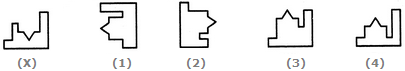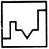# Non Verbal Reasoning - Shape Construction - Discussion

### Discussion :: Shape Construction - Section 3 (Q.No.9)

In each of the following questions, a key figure marked (X) is given, followed by four other alternative figures marked (1), (2), (3) and (4). It is required to select one figure from the alternatives, which fits exactly into figure (X) to form a complete square.

9.

Select a figure from the given four alternatives which fits exactly into Figure-X to form a complete square.[A]. 1 [B]. 2 [C]. 3 [D]. 4

Explanation:Raghu Prakash said: (Dec 20, 2016) I didn't understand, please can anybody explain this?

 Student said: (Nov 22, 2019) How is one and three different and how do you know which angle the shape should be in?